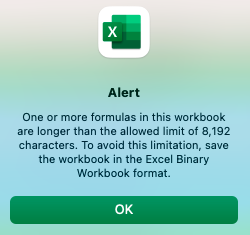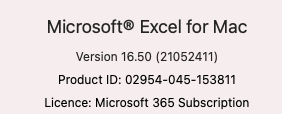troubleshooting Question

# How to find formula longer than 8192 chars in this workbook?asked on
Microsoft ExcelMicrosoft OfficeAnthony Mac

FOUND THIS:
Some formulas contain more values, references, and/or names than are supported by the selected file format. These formulas will not be saved and will be converted to #VALUE! errors.What it means    In Excel 2007 and later, the maximum length of formula contents is 8,192 characters, and the maximum internal formula length is 16,384 bytes. In Excel 97-2003, the maximum length of formula contents is 1,024 characters, and the maximum internal formula length is 1,800 bytes. When the combination of formula arguments (including values, references, and/or names) exceeds the maximum limits of Excel 97-2003, the formulas will result in #VALUE! errors when you save the workbook to an earlier Excel file format.
What to do    In the Compatibility Checker, click Find to locate the cells that contain formulas that exceed the maximum formula length limits of Excel 97-2003, and then make the necessary changes to avoid #VALUE! errors.
 Some formulas contain more values, references, and/or names than are supported by the selected file format. These formulas will not be saved and will be converted to #VALUE! errors. What it means    In Excel 2007 and later, the maximum length of formula contents is 8,192 characters, and the maximum internal formula length is 16,384 bytes. In Excel 97-2003, the maximum length of formula contents is 1,024 characters, and the maximum internal formula length is 1,800 bytes. When the combination of formula arguments (including values, references, and/or names) exceeds the maximum limits of Excel 97-2003, the formulas will result in #VALUE! errors when you save the workbook to an earlier Excel file format.What to do    In the Compatibility Checker, click Find to locate the cells that contain formulas that exceed the maximum formula length limits of Excel 97-2003, and then make the necessary changes to avoid #VALUE! errors.

SOLUTION
Robert Berke
Consultant
###### Learn from the best

Network and collaborate with thousands of CTOs, CISOs, and IT Pros rooting for you and your success.

Andrew Hancock - VMware vExpert
See if this solution works for you by signing up for a 7 day free trial.